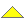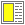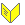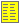Contracts and Assertions - slide 25 : 32

Class invariants in the triangle class hierarchy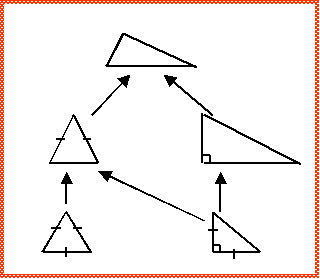Most general triangle: 3 non-zero angles 3 non-zero edges Sum of angles: 180 degrees Isosceles triangle Invariant of general triangle 2 edges of equal length Equilateral triangle: Invariant of isosceles triangle 3 edges of equal length Right triangle: Invariant of general triangle Pythagoras Isosceles right triangle: Invariant of isosceles triangle Invariant of right triangle